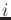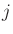# Rule base characteristics

The main characteristics of a rule base are summarized within a dedicated structure called InfoRB, the rule base information structure.

It is made up of the following attributes:

• In(i=1 to p) : number of MF for input• Out(j=1 to k) : number of possible (distinct) conclusions for output• Out : output number used for learning
• maxR : maximal number of rules according to the FIS structure
• nR : number of rules
• maxVr : maximum number of variables in a rule
• meanVr: mean number of variables per rule
• nVar : number of distinct variables used in rules
• meanMF: mean number of MF per used variable
• nClass: number of classes (0 if not classif)
• nRc : number of rules per class or MF

The structure also includes two methods allowing attribute handling: Print and WriteHeader to print the column labels. These print functions use as field separator the '&' character (Well known to latex users!)

The AnalyzeRB function, from class FIS, is used to fill a InfoRB structure with respect to a given output of a fuzzy inference system.